Request a Free Counselling Session from our Expert Mentor# NCERT Solution for Class 12 Physics Chapter 6

Welcome to the NCERT Solutions for Class 12 Physics Chapter 6, provided by Swastik Classes. In this chapter, you will learn about Electromagnetic Induction and Alternating Currents.

You will study the phenomenon of electromagnetic induction, which is the production of an electromotive force (emf) in a conductor due to a changing magnetic field. The chapter covers the concept of Faraday’s law of electromagnetic induction and Lenz’s law.

Additionally, the chapter discusses the concept of mutual induction, where the emf is induced in one coil due to the change of current in another coil. The chapter also covers the working principle of transformers, AC generators, and AC motors.

Our NCERT Solutions for Class 12 Physics Chapter 6 provide step-by-step explanations and solutions to all the questions in the textbook. With our solutions, you can easily understand the concepts covered in the chapter and develop a deeper understanding of the subject.

## Answers of Physics NCERT solutions for class 12 Chapter 6 – Electromagnetic Induction

Page No 229:

Question 6.1:

Predict the direction of induced current in the situations described by the following Figs. 6.18(a) to (f ).

(a)

(b)

(c)

(d)

(e)

(f)

The direction of the induced current in a closed loop is given by Lenz’s law. The given pairs of figures show the direction of the induced current when the North pole of a bar magnet is moved towards and away from a closed loop respectively.

Using Lenz’s rule, the direction of the induced current in the given situations can be predicted as follows:

(a) The direction of the induced current is along qrpq.

(b) The direction of the induced current is along prqp.

(c) The direction of the induced current is along yzxy.

(d) The direction of the induced current is along zyxz.

(e) The direction of the induced current is along xryx.

(f) No current is induced since the field lines are lying in the plane of the closed loop.

Page No 230:

Question 6.2:

Use Lenz’s law to determine the direction of induced current in the situations described by Fig. 6.19:

(a) A wire of irregular shape turning into a circular shape;

(b) A circular loop being deformed into a narrow straight wire.

According to Lenz’s law, the direction of the induced emf is such that it tends to produce a current that opposes the change in the magnetic flux that produced it.

(a) When the shape of the wire changes, the flux piercing through the unit surface area increases. As a result, the induced current produces an opposing flux. Hence, the induced current flows along adcb.

(b) When the shape of a circular loop is deformed into a narrow straight wire, the flux piercing the surface decreases. Hence, the induced current flows along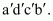Question 6.3:

A long solenoid with 15 turns per cm has a small loop of area 2.0 cmplaced inside the solenoid normal to its axis. If the current carried by the solenoid changes steadily from 2.0 A to 4.0 A in 0.1 s, what is the induced emf in the loop while the current is changing?

Number of turns on the solenoid = 15 turns/cm = 1500 turns/m

Number of turns per unit length, = 1500 turns

The solenoid has a small loop of area, A = 2.0 cm2 = 2 × 10−4 m2

Current carried by the solenoid changes from 2 A to 4 A.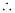Change in current in the solenoid, di = 4 − 2 = 2 A

Change in time, dt = 0.1 s

Induced emf in the solenoid is given by Faraday’s law as:

Where,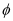= Induced flux through the small loop

BA … (ii)

= Magnetic field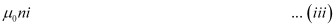μ0 = Permeability of free space

4π×10−7 H/m

Hence, equation (i) reduces to:

Hence, the induced voltage in the loop is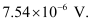Question 6.4:

A rectangular wire loop of sides 8 cm and 2 cm with a small cut is moving out of a region of uniform magnetic field of magnitude 0.3 T directed normal to the loop. What is the emf developed across the cut if the velocity of the loop is 1 cm s−1 in a direction normal to the (a) longer side, (b) shorter side of the loop? For how long does the induced voltage last in each case?

Length of the rectangular wire, l = 8 cm = 0.08 m

Width of the rectangular wire, b = 2 cm = 0.02 m

Hence, area of the rectangular loop,

A = lb

= 0.08 × 0.02

= 16 × 10−4 m2

Magnetic field strength, B = 0.3 T

Velocity of the loop, v = 1 cm/s = 0.01 m/s

(a) Emf developed in the loop is given as:

e = Blv

= 0.3 × 0.08 × 0.01 = 2.4 × 10−4 V

Hence, the induced voltage is 2.4 × 10−4 V which lasts for 2 s.

(b) Emf developed, e = Bbv

= 0.3 × 0.02 × 0.01 = 0.6 × 10−4 V

Hence, the induced voltage is 0.6 × 10−4 V which lasts for 8 s.

Question 6.5:

A 1.0 m long metallic rod is rotated with an angular frequency of 400 rad s−1 about an axis normal to the rod passing through its one end. The other end of the rod is in contact with a circular metallic ring. A constant and uniform magnetic field of 0.5 T parallel to the axis exists everywhere. Calculate the emf developed between the centre and the ring.

Length of the rod, l = 1 m

Magnetic field strength, B = 0.5 T

One end of the rod has zero linear velocity, while the other end has a linear velocity of lω.

Average linear velocity of the rod,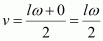Emf developed between the centre and the ring,

Hence, the emf developed between the centre and the ring is 100 V.

Question 6.6:

A circular coil of radius 8.0 cm and 20 turns is rotated about its vertical diameter with an angular speed of 50 rad s−1 in a uniform horizontal magnetic field of magnitude 3.0×10−2 T. Obtain the maximum and average emf induced in the coil. If the coil forms a closed loop of resistance 10Ω, calculate the maximum value of current in the coil. Calculate the average power loss due to Joule heating. Where does this power come from?

Max induced emf = 0.603 V

Average induced emf = 0 V

Max current in the coil = 0.0603 A

Average power loss = 0.018 W

(Power comes from the external rotor)

Radius of the circular coil, r = 8 cm = 0.08 m

Area of the coil, A = πr2 = π × (0.08)m2

Number of turns on the coil, N = 20

Angular speed, ω = 50 rad/s

Magnetic field strength, B = 3 × 10−2 T

Resistance of the loop, R = 10 Ω

Maximum induced emf is given as:

e = Nω AB

= 20 × 50 × π × (0.08)2 × 3 × 10−2

= 0.603 V

The maximum emf induced in the coil is 0.603 V.

Over a full cycle, the average emf induced in the coil is zero.

Maximum current is given as:

Average power loss due to joule heating:

The current induced in the coil produces a torque opposing the rotation of the coil. The rotor is an external agent. It must supply a torque to counter this torque in order to keep the coil rotating uniformly. Hence, dissipated power comes from the external rotor.

Question 6.7:

A horizontal straight wire 10 m long extending from east to west is falling with a speed of 5.0 m s−1, at right angles to the horizontal component of the earth’s magnetic field, 0.30 × 10−4 Wb m−2.

(a) What is the instantaneous value of the emf induced in the wire?

(b) What is the direction of the emf?

(c) Which end of the wire is at the higher electrical potential?

Length of the wire, l = 10 m

Falling speed of the wire, v = 5.0 m/s

Magnetic field strength, B = 0.3 × 10−4 Wb m−2

(a) Emf induced in the wire,

e = Blv

(b) Using Fleming’s right hand rule, it can be inferred that the direction of the induced emf is from West to East.

(c) The eastern end of the wire is at a higher potential.

Question 6.8:

Current in a circuit falls from 5.0 A to 0.0 A in 0.1 s. If an average emf of 200 V induced, give an estimate of the self-inductance of the circuit.

Initial current, I1 = 5.0 A

Final current, I2 = 0.0 A

Change in current,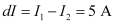Time taken for the change, t = 0.1 s

Average emf, e = 200 V

For self-inductance (L) of the coil, we have the relation for average emf as:

e = L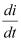Hence, the self induction of the coil is 4 H.

Question 6.9:

A pair of adjacent coils has a mutual inductance of 1.5 H. If the current in one coil changes from 0 to 20 A in 0.5 s, what is the change of flux linkage with the other coil?

Mutual inductance of a pair of coils, µ = 1.5 H

Initial current, I1 = 0 A

Final current I2 = 20 A

Change in current,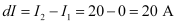Time taken for the change, t = 0.5 s

Induced emf,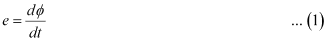Where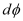is the change in the flux linkage with the coil.

Emf is related with mutual inductance as:

Equating equations (1) and (2), we get

Hence, the change in the flux linkage is 30 Wb.

Question 6.10:

A jet plane is travelling towards west at a speed of 1800 km/h. What is the voltage difference developed between the ends of the wing having a span of 25 m, if the Earth’s magnetic field at the location has a magnitude of 5 × 10−4 T and the dip angle is 30°.

Speed of the jet plane, v = 1800 km/h = 500 m/s

Wing spanof jet plane, l = 25 m

Earth’s magnetic field strength, B = 5.0 × 10−4 T

Angle of dip,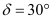Vertical component of Earth’s magnetic field,

BV = B sin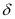= 5 × 10−4 sin 30°

= 2.5 × 10−4 T

Voltage difference between the ends of the wing can be calculated as:

e = (BV) × l × v

= 2.5 × 10−4 × 25 × 500

= 3.125 V

Hence, the voltage difference developed between the ends of the wings is

3.125 V.

Page No 231:

Question 6.11:

Suppose the loop in Exercise 6.4 is stationary but the current feeding the electromagnet that produces the magnetic field is gradually reduced so that the field decreases from its initial value of 0.3 T at the rate of 0.02 T s−1. If the cut is joined and the loop has a resistance of 1.6 Ω how much power is dissipated by the loop as heat? What is the source of this power?

Sides of the rectangular loop are 8 cm and 2 cm.

Hence, area of the rectangular wire loop,

A = length × width

= 8 × 2 = 16 cm2

= 16 × 10−4 m2

Initial value of the magnetic field,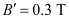Rate of decrease of the magnetic field,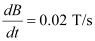Emf developed in the loop is given as:

Where,= Change in flux through the loop area

AB

Resistance of the loop, R = 1.6 Ω

The current induced in the loop is given as:

Power dissipated in the loop in the form of heat is given as:

The source of this heat loss is an external agent, which is responsible for changing the magnetic field with time.

Question 6.12:

A square loop of side 12 cm with its sides parallel to X and Y axes is moved with a velocity of 8 cm s−1 in the positive x-direction in an environment containing a magnetic field in the positive z-direction. The field is neither uniform in space nor constant in time. It has a gradient of 10−3 T cm−1 along the negative x-direction (that is it increases by 10− 3 T cm−1 as one moves in the negative x-direction), and it is decreasing in time at the rate of 10−3 T s−1. Determine the direction and magnitude of the induced current in the loop if its resistance is 4.50 mΩ.

Side of the square loop, s = 12 cm = 0.12 m

Area of the square loop, A = 0.12 × 0.12 = 0.0144 m2

Velocity of the loop, v = 8 cm/s = 0.08 m/s

Gradient of the magnetic field along negative x-direction,

And, rate of decrease of the magnetic field,

Resistance of the loop,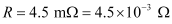Rate of change of the magnetic flux due to the motion of the loop in a non-uniform magnetic field is given as:

Rate of change of the flux due to explicit time variation in field is given as:

Since the rate of change of the flux is the induced emf, the total induced emf in the loop can be calculated as:

∴Induced current,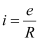Hence, the direction of the induced current is such that there is an increase in the flux through the loop along positive z-direction.

Question 6.13:

It is desired to measure the magnitude of field between the poles of a powerful loud speaker magnet. A small flat search coil of area 2 cm2 with 25 closely wound turns, is positioned normal to the field direction, and then quickly snatched out of the field region. Equivalently, one can give it a quick 90° turn to bring its plane parallel to the field direction). The total charge flown in the coil (measured by a ballistic galvanometer connected to coil) is 7.5 mC. The combined resistance of the coil and the galvanometer is 0.50 Ω. Estimate the field strength of magnet.

Area of the small flat search coil, A = 2 cm= 2 × 10−4 m2

Number of turns on the coil, N = 25

Total charge flowing in the coil, Q = 7.5 mC = 7.5 × 10−3 C

Total resistance of the coil and galvanometer, R = 0.50 Ω

Induced current in the coil,

Induced emf is given as:

Where,= Charge in flux

Combining equations (1) and (2), we get

Initial flux through the coil,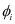= BA

Where,

B = Magnetic field strength

Final flux through the coil,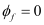Integrating equation (3) on both sides, we have

But total charge,Hence, the field strength of the magnet is 0.75 T.

Question 6.14:

Figure 6.20 shows a metal rod PQ resting on the smooth rails AB and positioned between the poles of a permanent magnet. The rails, the rod, and the magnetic field are in three mutual perpendicular directions. A galvanometer G connects the rails through a switch K. Length of the rod = 15 cm, = 0.50 T, resistance of the closed loop containing the rod = 9.0 mΩ. Assume the field to be uniform.

(a) Suppose K is open and the rod is moved with a speed of 12 cm s−1 in the direction shown. Give the polarity and magnitude of the induced emf.

(b) Is there an excess charge built up at the ends of the rods when

K is open? What if K is closed?

(c) With K open and the rod moving uniformly, there is no net force on the electrons in the rod PQ even though they do experience magnetic force due to the motion of the rod. Explain.

(d) What is the retarding force on the rod when K is closed?

(e) How much power is required (by an external agent) to keep the rod moving at the same speed (=12 cm s−1) when K is closed? How much power is required when K is open?

(f) How much power is dissipated as heat in the closed circuit?

What is the source of this power?

(g) What is the induced emf in the moving rod if the magnetic field is parallel to the rails instead of being perpendicular?

Length of the rod, l = 15 cm = 0.15 m

Magnetic field strength, B = 0.50 T

Resistance of the closed loop, R = 9 mΩ = 9 × 10−3 Ω

(a) Induced emf = 9 mV; polarity of the induced emf is such that end P shows positive while end Q shows negative ends.

Speed of the rod, v = 12 cm/s = 0.12 m/s

Induced emf is given as:

e = Bvl

0.5 × 0.12 × 0.15

= 9 × 10−3 v

= 9 mV

The polarity of the induced emf is such that end P shows positive while end Q shows negative ends.

(b) Yes; when key K is closed, excess charge is maintained by the continuous flow of current.

When key K is open, there is excess charge built up at both ends of the rods.

When key K is closed, excess charge is maintained by the continuous flow of current.

(c) Magnetic force is cancelled by the electric force set-up due to the excess charge of opposite nature at both ends of the rod.

There is no net force on the electrons in rod PQ when key K is open and the rod is moving uniformly. This is because magnetic force is cancelled by the electric force set-up due to the excess charge of opposite nature at both ends of the rods.

(d) Retarding force exerted on the rod, F = IBl

Where,

I = Current flowing through the rod

(e) 9 mW; no power is expended when key K is open.

Speed of the rod, v = 12 cm/s = 0.12 m/s

Hence, power is given as:

When key K is open, no power is expended.

(f) 9 mW; power is provided by an external agent.

Power dissipated as heat = I2 R

= (1)2 × 9 × 10−3

= 9 mW

The source of this power is an external agent.

(g) Zero

In this case, no emf is induced in the coil because the motion of the rod does not cut across the field lines.

Page No 232:

Question 6.15:

An air-cored solenoid with length 30 cm, area of cross-section 25 cm2 and number of turns 500, carries a current of 2.5 A. The current is suddenly switched off in a brief time of 10−3 s. How much is the average back emf induced across the ends of the open switch in the circuit? Ignore the variation in magnetic field near the ends of the solenoid.

Length of the solenoid, l = 30 cm = 0.3 m

Area of cross-section, A = 25 cm2 = 25 × 10−4 m2

Number of turns on the solenoid, N = 500

Current in the solenoid, I = 2.5 A

Current flows for time, t = 10−3 s

Average back emf,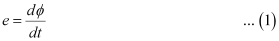Where,= Change in flux

NAB … (2)

Where,

B = Magnetic field strength

Where,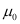= Permeability of free space = 4π × 10−7 T m A−1

Using equations (2) and (3) in equation (1), we get

Hence, the average back emf induced in the solenoid is 6.5 V.

Question 6.16:

(a) Obtain an expression for the mutual inductance between a long straight wire and a square loop of side as shown in Fig. 6.21.

(b) Now assume that the straight wire carries a current of 50 A and the loop is moved to the right with a constant velocity, = 10 m/s.

Calculate the induced emf in the loop at the instant when = 0.2 m.

Take = 0.1 m and assume that the loop has a large resistance.

(a) Take a small element dy in the loop at a distance y from the long straight wire (as shown in the given figure).

Magnetic flux associated with element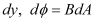Where,

dA = Area of element dy a dy

B = Magnetic field at distance y

I = Current in the wire= Permeability of free space = 4π × 10−7 T m A−1

y tends from x to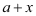.

(b) Emf induced in the loop, e = B’av

Given,

= 50 A

x = 0.2 m

a = 0.1 m

v = 10 m/s

Question 6.17:

A line charge λ per unit length is lodged uniformly onto the rim of a wheel of mass and radius R. The wheel has light non-conducting spokes and is free to rotate without friction about its axis (Fig. 6.22). A uniform magnetic field extends over a circular region within the rim. It is given by,

B = − B0 k (≤ aR)

= 0 (otherwise)

What is the angular velocity of the wheel after the field is suddenly switched off?

Line charge per unit length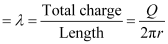Where,

= Distance of the point within the wheel

Mass of the wheel = M

Radius of the wheel = R

Magnetic field,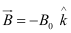At distance r,themagnetic force is balanced by the centripetal force i.e.,

∴Angular velocity,## Class 12 Physics NCERT Solutions for Chapter 6 – Electromagnetic Induction

As discussed earlier in Class 12 Chapter 4 of the NCERT, electricity, and magnetism were considered to be separate phenomena until around the mid-1800s. The contemporary experiments conducted by Michael Faraday and Joseph Henry around 1830 revealed that electricity and magnetism were interconnected somehow. Faraday displayed through his first public experiment that a relative motion between an electric conductor and a magnet induced electric current in the conductor. Our world as we know it owes a great deal to the discoveries made by these two scientists. Modern-day transformers and appliances widely see an application of the laws of Electromagnetic Induction. NCERT Class 12 Physics Chapter 6 deals with the Laws of Electromagnetic Induction, its nuances, and its applications.

### Why do students prefer SWC’s NCERT Solutions for Physics Class 12 Chapter 6?

At SWC, students directly learn from IITians and experienced faculties. We provide quality study materials to prepare and follow a LEAP model. We teach not only for exams but for lifelong applications.## FAQs on Physics Class 12 NCERT Chapter 6 – Electromagnetic Induction

### What does emf mean in physics?

In an open circuit/when no current flows, Emf is equal to the terminal potential difference.

### Why emf is called force?

Alessandro Volta coined the term “Electromotive Force”. He stated that there existed a force that separated the charges in current flowing through a closed circuit. This name coined by him remained even after the meaning of the term was refined.

### Is emf equal to voltage?

Only when there is no current flowing in the circuit.

### What is the principle of a potentiometer?

The principle of a potentiometer is that the potential dropped across a segment of a wire of uniform cross-section carrying a constant current is directly proportional to its length.

### What is self-inductance?

Self-Inductance is the tendency of a coil to reset any change in the current flowing through it.

Yes

### What is the limitation of Ohm’s law?

Ohm’s law does not apply to unilateral or non-linear elements with constraints like capacitance.

## Summary of NCERT Solutions for Class 12 Physics Chapter 6

• The process of creating an electric current with a magnetic field is known as electromagnetic induction. When a magnetic field and an electric conductor move relative to one another, the conductor crosses the magnetic field’s lines of force.
• When the magnet or coil moves faster, the coil has more turns, or the magnet is stronger, the current produced by electromagnetic induction increases. Alternating current is created when a magnet or coil is repeatedly pushed back and forth.
• Electromagnetic induction is used by electric generators and electric transformers to create electricity or adjust the voltage of electric current.

Conclusion

The NCERT Solutions for Class 12 Physics Chapter 6 provided by Swastik Classes offer a comprehensive understanding of the concepts of Electromagnetic Induction and Alternating Currents. The solutions provide step-by-step explanations of all the questions given in the textbook, making it easier for students to grasp the concepts and solve the problems with ease.

Our solutions cover all the important topics of the chapter, such as Faraday’s law of electromagnetic induction, Lenz’s law, mutual induction, self-induction, transformers, AC generators, and AC motors. Furthermore, the solutions provide practical applications of these concepts in various fields, such as power generation, transmission, and distribution.

With these solutions, students can develop a better understanding of the subject and excel in their exams. The solutions are designed to help students gain a deeper understanding of the concepts covered in the chapter and provide them with the necessary tools to solve complex problems.

Overall, the NCERT Solutions for Class 12 Physics Chapter 6 are an excellent resource for students who want to develop a strong foundation in the subject of Electromagnetic Induction and Alternating Currents. With these solutions, students can improve their problem-solving skills and achieve academic success.